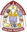Trending ▼   ResFinder# ICSE Prelims 2018 : Mathematics (Maneckji Cooper Education Trust School, Mumbai)

4 pages, 54 questions, 49 questions with responses, 164 total responses,20Tanish Shah Children's Academy, Bachani Nagar, Malad East, Mumbai 5th to 10th
+Fave Message
 Home > tanish02 >   F Also featured on: School Page icseFormatting page ...

t u A * in o . \ U ln b so . f tu B h m U e m = r ln o m e u e o f i u e w d o d t c c o p u d , h e n m n l i d l m lc m a # . r d h & c n M Q 2 3 1 0 0 V A T H S le p ie o f b c U m o u n t v A T i) ii) iii) P r ic e im P c r i s e c io . _ T n - x m l lx r m - 1 2 t l , o r 5 y - r be . u t 4 332o n n u m o f W q a o o t o f r o d cr w to p e il e r m r t v A T A ) a C p C u p t q d o f r m 2 - - F i d 0 % , in a n * o p iw p id h ( 2 3 10 0 n m er m W V A T i o c o m . u m a 1 h c w h t o k 2 6 o o o l c r . n f d lu c - n ph a d u h b e c u r to m p id h r r h m m i h c n n . er 4 . = h l 2x 1 5 R I 3 s . 4 x < t n g e o f e t p x 23 n . = q C n d w i o c m m b l in c 3 \ Scanned by CamScannerFormatting page ...

Top Contributors
to this ResPaperAayush Patel(24)Smit Shah(22)M_E_D_I N_B_(21)Atharva Malgundkar(20)Formatting page ...Formatting page ...